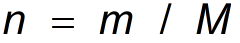Request a Tool

Grams to Moles Calculator

Divide the number of Mass in grams by the molar mass

Number of Moles
0

Formula• n = Number of Moles
• m = Mass
• Molar Mass = 18.015

Defination / Uses

Mole is a common scientific unit for measuring large quantities of very small things such as atoms, molecules, or other designated particles in chemistry.

The mole signifies 6.02214076 1023 units, which is an extremely big quantity. In the metric system, a gramme is defined as one thousandth (1 x 10-3) of a kilogramme.

Example

Find the amount of moles by converting grammes to moles. Water Molar Mass is Equal to 18.015g/mol, mass is 20 gram. Number of Moles is 1.11 mol.